# What is the integrationof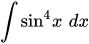?Melina

Solution:

Concept:The integration of a function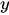is the reciprocal of derivative of the function. It is denoted by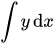.

The anti-derivative of some functionsare given in the table below: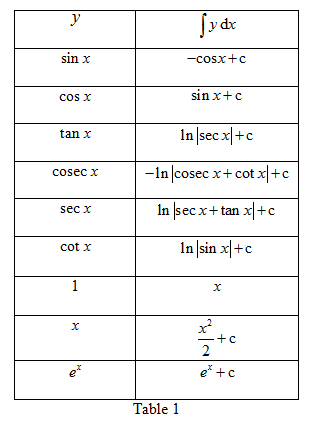Calculation:

Step:1 The function to be integrated is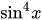.Step:2 From the Table 1, the integration expression is solved by using the integration of constant value 1 as x and integration of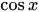as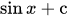.

Step:3 Hence, the integration ofis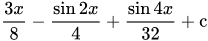.

Answer:The value of integrationis.

Similar Problems:

Problem 1:What is anti-derivative of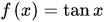?

Solution:

Step:1 From the Table 1, it is observed the anti-derivative of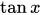is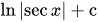.

Since anti-derivative is the reciprocal of derivative, the derivative ofmust be.

Verify the result by taking derivative ofas shown below:

Let the function be y such that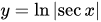.

Step:2 Now take the derivative of the above equation.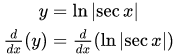Step:3 Simplify further to solve for the derivate as shown below:Hence, the result obtained from the table is verified and anti-derivative ofis.

Answer:The anti-derivative ofis.

Problem 2:What is anti-derivative of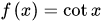?

Solution:

Step:1  From the Table 1, it is observed the anti-derivative ofis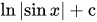.

Since anti-derivative is the reciprocal of derivative, the derivative ofmust be.

Verify the result by taking derivative ofas shown below:

Let the function be y such that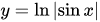.

Step:2 Now take the derivative of the above equation.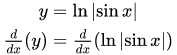Step:3 Simplify further to solve for the derivate as shown below:Hence, the result obtained from the table is verified and anti-derivative ofis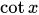\.

Answer: The anti-derivative ofis.

### Need homework help now?

tutors online

Related Questions
##### (t) in °C of the pot of soup at time t (in The initial temperature of a pot of soup is 80°C. The temperature minutes) satisfies the differential equation dT di T-20 10 (a) Solve the differential equation, and find an explicit expression for the function (). By separating variables, integrating and manipulating arbitrary constants, . We are also told that so that and so . ...
Notes
Me

OR

Don't have an account?

Join OneClass

Access over 10 million pages of study
documents for 1.3 million courses.

Join to view

OR

By registering, I agree to the Terms and Privacy Policies
Just a few more details

So we can recommend you notes for your school.